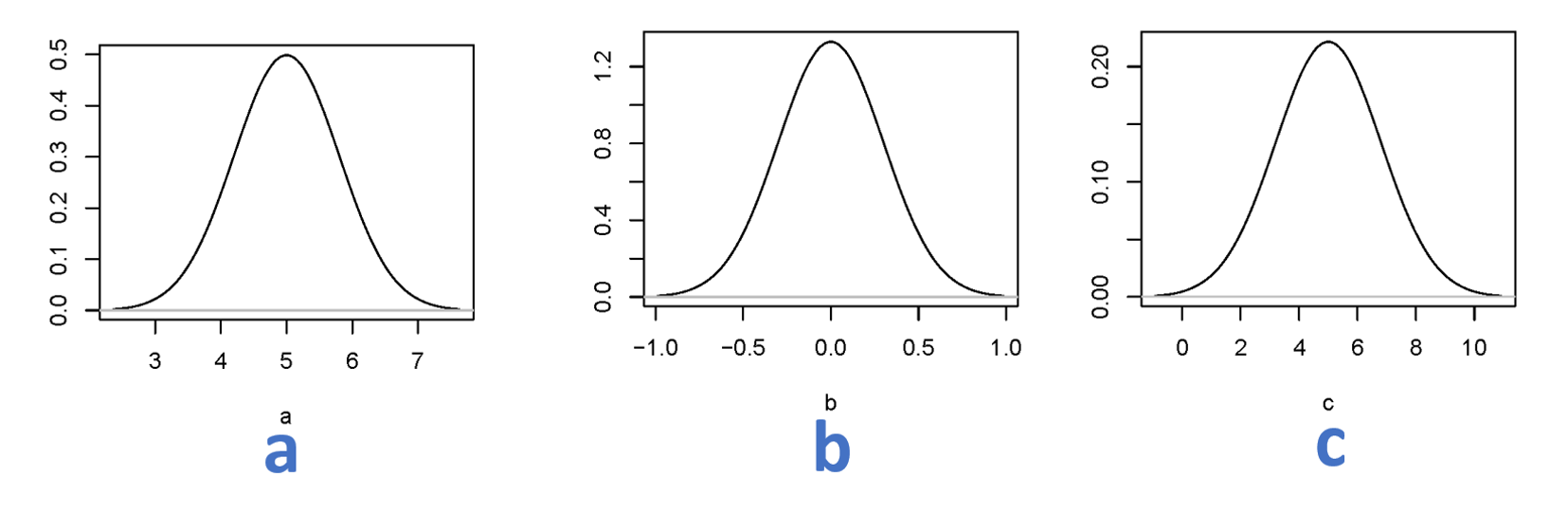0
Correct
0
Incorrect
7
Total

## Question 1 of 7

The life time of batteries purchased from two different producers is investigated. The lifetime of a number of batteries from each manufacturer is recorded and the following data is available:

Producer A: $${n_A} = 15\quad \left( { { {\bar x}_A},{s_A}} \right) = \left( {122.4;30.5} \right)$$

Producer B: $${n_B} = 10\quad \left( { { {\bar x}_B},{s_B}} \right) = \left( {145.9;22.3} \right)$$

Data is assumed to follow a normal distribution in each group. What is the test statistic for and the conclusion about the hypothesis ${\mu_A} = {\mu}_B$: (Both $t$-value and the conclusion must be valid)

## Question 2 of 7

Data in the table below comes from a study where 14 patient’s systolic blood pressure was measured (in mmHg) before a certain medical drug treatment and 1 month after starting the treatment.

Patient no 1 2 3 4 5 6 7 8 9 10 11 12 13 14
Blood pressure before treatment 155 164 177 167 156 198 155 187 185 177 150 145 189 176
Blood pressure after treatment 165 154 172 156 150 185 145 182 165 166 145 150 169 172

A 95% confidence interval for the change in blood pressure becomes:

## Question 3 of 7

In a sports study one wants to investigate whether there is a difference in energy consumption for various types of training. We have (for a single person) measured the energy consumed in 10 jogs of 30 minutes and 10 bike rides of 30 minutes. Measurements, expressed in kcal, is given in the table below:

Jogs Bike rides
314 294
340 317
331 317
333 310
329 327
322 300
332 293
330 321
338 307
325 304

The following R code was run:

	x1=c(314,340,331,333,329,322,332,330,338,325)
x2=c(294,317,317,310,327,300,293,321,307,304)
var(x1)
var(x2)
t.test(x1,x2)
t.test(x1,x2,pair=T,mu=20)


with the following results:

> var(x1)
 57.82222
> var(x2)
 132
> t.test(x1,x2)

Welch Two Sample t-test

data:  x1 and x2
t = 4.6823, df = 15.615, p-value = 0.0002658
alternative hypothesis: true difference in means is not equal to 0
95 percent confidence interval:
11.14535 29.65465
sample estimates:
mean of x mean of y
329.4     309.0

> t.test(x1,x2,pair=T,mu=20)

Paired t-test

data:  x1 and x2
t = 0.1209, df = 9, p-value = 0.9064
alternative hypothesis: true difference in means is not equal to 20
95 percent confidence interval:
12.91852 27.88148
sample estimates:
mean of the differences
20.4


Consider only data for the bike rides. Denote the true mean energy consumption by bike rides $\mu$. A 99% confidence interval for $\mu$ is:

## Question 4 of 7

If you did the previous exercise, the following is a repetition: In a sports study one wants to investigate whether there is a difference in energy consumption for various types of training. We have (for a single person) measured the energy consumed in 10 jogs of 30 minutes and 10 bike rides of 30 minutes. Measurements, expressed in kcal, is given in the table below:

Jogs Bike rides
314 294
340 317
331 317
333 310
329 327
322 300
332 293
330 321
338 307
325 304

The following R code was run:

	x1=c(314,340,331,333,329,322,332,330,338,325)
x2=c(294,317,317,310,327,300,293,321,307,304)
var(x1)
var(x2)
t.test(x1,x2)
t.test(x1,x2,pair=T,mu=20)


with the following results:

> var(x1)
 57.82222
> var(x2)
 132
> t.test(x1,x2)

Welch Two Sample t-test

data:  x1 and x2
t = 4.6823, df = 15.615, p-value = 0.0002658
alternative hypothesis: true difference in means is not equal to 0
95 percent confidence interval:
11.14535 29.65465
sample estimates:
mean of x mean of y
329.4     309.0

> t.test(x1,x2,pair=T,mu=20)

Paired t-test

data:  x1 and x2
t = 0.1209, df = 9, p-value = 0.9064
alternative hypothesis: true difference in means is not equal to 20
95 percent confidence interval:
12.91852 27.88148
sample estimates:
mean of the differences
20.4


A new study in energy consumption during by bike rides is planned. We would like a 95\% confidence interval for $\mu$ with a total width of $8$ kcal. How many bike rides should we approximately carry out to achieve this precision?

## Question 5 of 7

The probability density functions of three normal distributions are shown below:It is given that the standard deviation of the distribution in the middle is $\sigma = 0.3$. If you have to mention the three distributions in order after the size of the standard deviation, where you mention the distribution with the lowest standard deviation first and the distribution with the largest standard deviation at last, the correct order is?

## Question 6 of 7

In order for a taxi company to make money, the number of customers for a given taxi driver should at least be 4 per. hour. In a period of 3 hours, 9 costumers were observed at a random selected taxi driver. It is desired to investigate whether there is reason to believe that the taxi company lose money on this taxi driver in the long run?

The $p$-value for such a test is given by:

## Question 7 of 7

A strength calculation on an old tube in a construction is to be performed. Because of corrosion and age diameters are quite ‘indeterminate’. Therefore several measurements are made of as well outer as inner diameter. The measurements of outer respectively inner diameter are independent of each other. The results are listed below: (all dimensions in mm)

Outer diameter, x: 44.9, 44.2 , 44.6, 44.8 , 44.0, 45.1

Inner diameter, y: 32.4, 32.5, 31.5, 32.2, 32.6, 31.7

$\left( {\bar x;{s_x}} \right) = (44.6;0.424)\,\mathrm{mm}\quad \mbox{and} \quad \left({\bar y;{s_y}} \right) = (32.15;0.451)\,\mathrm{mm}$

Assume that the standard deviation of the measurements on the outer diameter is $\sigma_x = 0.43$. A new study is planned in which the outer diameter should be determined with an accuracy corresponding to a 95% confidence interval of size $\pm$ 0.1.

What number of measurements, $n$ is required?